# Area + cylinder - math problems

#### Number of problems found: 86

• Cylinder surface, volumeThe area of the cylinder surface and the cylinder jacket are in the ratio 3: 5. The height of the cylinder is 5 cm shorter than the radius of the base. Calculate surface area and volume of the cylinder.
• The cylinderThe cylinder has a surface area of 300 square meters, while the height of the cylinder is 12 m. Calculate the volume of this cylinder.
• Cylinder - areaThe diameter of the cylinder is one-third the length of the height of the cylinder. Calculate the surface of cylinder if its volume is 2 m3.
• Cylinder - A&VThe cylinder has a volume 1287. The base has a radius 10. What is the area of surface of the cylinder?
• Cylinder surface areaVolume of a cylinder whose height is equal to the radius of the base is 678.5 dm3. Calculate its surface area.
• Rotary cylinder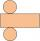The rotating cylinder has a surface area 69.08 cm2. The area of the shell is 62.8 cm 2. What is the diameter of the cylinder?
• Rotary cylinder 2Base circumference of the rotary cylinder has same length as its height. What is the surface area of cylinder if its volume is 250 dm3?
• Volume and surfaceCalculate the volume and surface area of the cylinder when the cylinder height and base diameter is in a ratio of 3:4 and the area of the cylinder jacket is 24 dm2.
• RollerCylinder shell has the same content as one of its bases. Cylinder height is 15 dm. What is the radius of the base of the cylinder?
• Cylinder diameter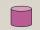The surface of the cylinder is 149 cm2. The cylinder height is 6 cm. What is the diameter of this cylinder?
• Shell area cyThe cylinder has a shell content of 300 cm square, while the height of the cylinder is 12 cm. Calculate the volume of this cylinder.
• Cylinder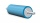The 1.8m cylinder contains 2000 liters of water. What area (in dm2) of this container is the water?
• Surface of the cylinderCalculate the surface area of the cylinder when its volume is 45 l and the perimeter of base is three times of the height.
• The cylinder 2Find the volume and the lateral area of a cylinder of height 12 inches and a base radius of 4 inches.
• Equilateral cylinderEquilateral cylinder (height = base diameter; h = 2r) has a volume of V = 199 cm3 . Calculate the surface area of the cylinder.
• Cylinder melted into cuboid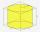A circular cylinder has area of cross section 56cm2 and the height is 10cm the cylinder is melted and made into a cuboid of base area 16cm2. What is the height of the cuboid?
• Surface area of cylinder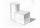Determine the lateral surface of the rotary cylinder which is circumscribed cube with edge length 5 cm.
• Cylinder - h2Cylinder volume is 2.6 liters. Base area is 1.3 dm2. Calculate the height of the cylinder.
• Axial sectionThe axial section of the cylinder has a diagonal 31 cm long, and we know that the area of the side and the base area is in ratio 3:2. Calculate the height and radius of the cylinder base.
• Cylinder - basicsCylinder with base radius r = 45 dm and height h=22 dm. Calculate:

Do you have an interesting mathematical word problem that you can't solve it? Submit a math problem, and we can try to solve it.

We will send a solution to your e-mail address. Solved examples are also published here. Please enter the e-mail correctly and check whether you don't have a full mailbox.

Please do not submit problems from current active competitions such as Mathematical Olympiad, correspondence seminars etc...

Area - math word problems. Cylinder Problems.